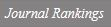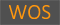TRACE EXPRESSION OF r-TH ROOT OVER FINITE FIELD

Title
TRACE EXPRESSION OF r-TH ROOT OVER FINITE FIELD
Authors
Cho, Gook HwaKoo, NamhunKwon, Soonhak
Ewha Authors
Issue Date
2020
Journal Title
JOURNAL OF THE KOREAN MATHEMATICAL SOCIETY
ISSN
0304-99142234-3008Citation
JOURNAL OF THE KOREAN MATHEMATICAL SOCIETY vol. 57, no. 4, pp. 1019 - 1030
Keywords
Finite fieldtracer-th rootlinear recurrence relationTonelli-Shanks algorithmAdleman-Manders-Miller algorithmCipolla-Lehmer algorithm
Publisher
KOREAN MATHEMATICAL SOC
Indexed
SCIE; SCOPUS; KCIDocument Type
Article
Abstract
Efficient computation of r-th root in F-q has many applications in computational number theory and many other related areas. We present a new r-th root formula which generalizes Muller's result on square root, and which provides a possible improvement of the CipollaLehmer type algorithms for general case. More precisely, for given r-th power c is an element of F-q, we show that there exists alpha is an element of Fq(r)>such that Tr(alpha(Sigma i=0r-1 qi)-r/r2)(r) = c, where Tr (alpha) = alpha + alpha(q) + alpha(q2) + . . . + a(qr-1) and a is a root of certain irreducible polynomial of degree r over F-q.
DOI
10.4134/JKMS.j190569
Appears in Collections:
연구기관 > 수리과학연구소 > Journal papers
Files in This Item:
There are no files associated with this item.
Export
RIS (EndNote)
XLS (Excel)
XML